# Fixing the p-value note in a HTML stargazer table

If you insert a stargazer table into R Markdown that outputs an HTML file, you may get a p-value note that looks like this:

p<0.1; p<0.05; p<0.01

What should be displayed is this:

*p<0.1; **p<0.05; ***p<0.01

This is the legend for understanding the “stars” in the regression table. What’s happening is the asterisks are being treated as markdown code, which is adding italics and bolding to the note instead of simply showing the asterisks verbatim. (Recall that in Markdown surrounding text with one asterisk adds italics and surrounding text with two asterisks adds bolding.)

To fix this we can add the following two arguments to the stargazer function:

notes.append = FALSE

notes = c("<sup>&sstarf;</sup>p<0.1; <sup>&sstarf;&sstarf;</sup>p<0.05; <sup>&sstarf;&sstarf;&sstarf;</sup>p<0.01")

&sstarf; is an HTML entity for a star character. notes.append = FALSE says to NOT append the note but rather replace the existing note. The notes argument specifies the note we want to add using the &sstarf; HTML entity.

# Recreating a Geometric CDF plot from Casella and Berger

For reasons I no longer remember I decided a while back to reproduce Figure 1.5.2 from Casella and Berger (page 32):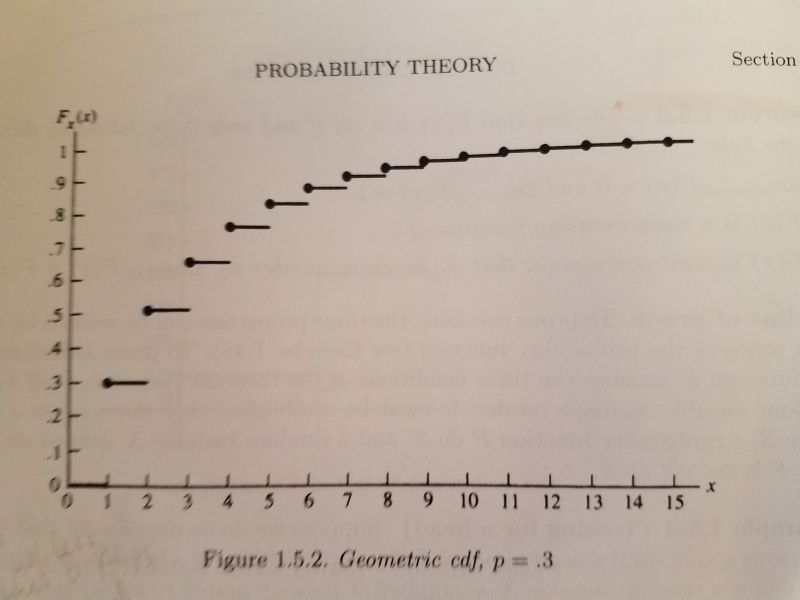It's a plot of the cumulative distribution function of a geometric distribution with p = 0.3. The geometric distribution is best explained with coin-flipping. Let's say we keep flipping a coin until we observe a head. Now let X be a random variable that equals the number of tosses required to see a head. So if we get a head on the first flip of the coin, that means we needed one toss. If it takes two tosses, we got a tail on the first toss and then a head on the second toss. The graph above visualizes the possibilities for 15 flips with probability of getting heads set to 0.3. We see that the probability of taking only one toss is 0.3, which makes sense since the probability of getting heads is 0.3. The probability of requiring two tosses is about 0.5. And so on. The straight lines indicate the probabilities only change at the whole numbers. There is no such thing as, say, 2.23 flips. This is often referred to as a step function. We see that there's a high probability of getting a head by the 5th or 6th flip. This is a simple waiting-time distribution that can be used to model the number of successes before a failure and vice-versa.

To recreate this plot in R I used the pgeom function, which returns the cumulative probability of the geometric distribution for a given number of failures before a success occurs and a given probability of success. For example, the probability of 0 failures before observing a success with p = 0.3:

pgeom(0, prob = 0.3)

##  0.3


The probability of 1 failure before observing a success with p = 0.3:

pgeom(1, prob = 0.3)

##  0.51


To generate all the probabilities in the plot, we can do the following:

y <- pgeom(q = 0:14, prob = 0.3)


Notice that instead of using 1:15 (number of total flips for success), we use 0:14. That's because pgeom works with the number of failures before success. Now that we have our y coordinates, we can create our first version of the plot as follows:

plot(x = 1:15, y, pch = 19)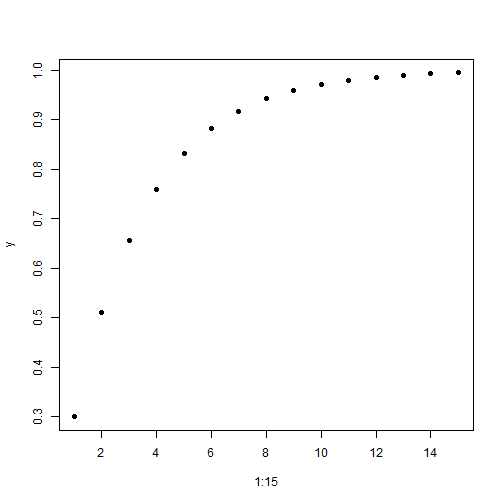Now let's add the horizontal lines using the segments function:

plot(x = 1:15, y, pch = 19)
segments(x0 = 0:15, y0 = c(0, y),
x1 = 1:16, y1 = c(0, y), lwd = 2)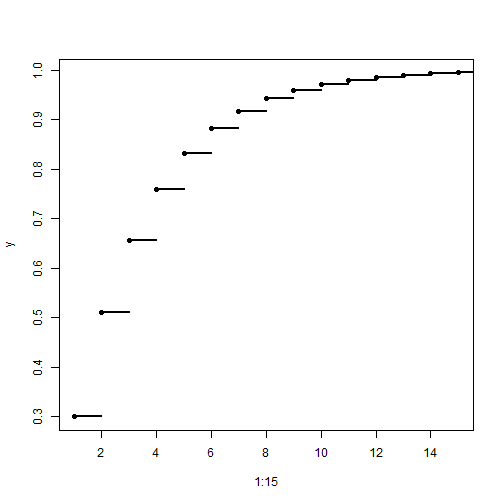The x0 and y0 coordinates are where the line starts. The x1 and y1 coordinates are where the line ends. Since the lines are horizontal, the y coordinates are the same for the start and end postions. The y coordinates include 0, so we add that value to y with the c function. The lwd = 2 argument makes the line a little thicker and darker.

Our plot is basically done, but just for fun I wanted to see how close I could make it look like the version in the book. That means relabeling the axes, moving the axis labels to the ends, and removing the lines at the top and right side of the plot. It also means moving the axis tick marks inside the plotting area. After some trial and error and deep dives into R documentation, here's what I was able to come up with:

plot(x = 1:15, y, pch = 19,
yaxt = "n", xaxt = "n", ylim = c(0,1.05), xlim = c(0,15.5),
bty="l", xaxs="i", yaxs = "i", xlab = "", ylab = "")
segments(x0 = 0:15, y0 = c(0, y),
x1 = 1:16, y1 = c(0, y), lwd = 2)
axis(side = 1, at = 0:15,
labels = 0:15, tcl = 0.5, family = "serif")
axis(side = 2, at = seq(0,1,0.1),
labels = c(0,paste(".",1:9),1), las=1, tcl = 0.5, family = "serif")
mtext(text = expression(italic(x)), side = 4,
at = 0, las = 1, line = 0.5, family = "serif")
mtext(text = expression(italic(F[x](x))), side = 3,
at = 0, line = 0.5, family = "serif")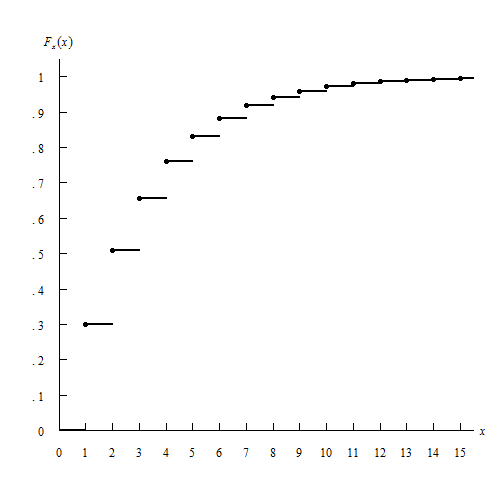In the plot function:

• the yaxt = "n" and xaxt = "n" arguments say “don't label the axes”. I instead use the axis function to create the axes.
• the ylim = c(0,1.05) and xlim = c(0,15.5) arguments tell the axes to end at 1.05 and 15.5, respectively. I wanted them to extend beyond the last value just as they do in the book.
• the bty="l" argument says “draw a box around the plot like a capital letter L”
• the xaxs="i" and yaxs = "i" arguments ensures the axes fit within the original range of the data. The default is to extend the range by 4 percent at each end. Again, I was trying to recreate the graph in the book. Notice how the origin has the x-axis and y-axis 0 values right next to one another.
• The xlab = "" and ylab = "" set blank axis labels. I instead use the mtext function to add axis labels.

The segments function remained unchanged.

The axis function allows us to explicitly define how the axis is created.

• The side argument specifies which side of the plot we're placing the axis. 1 is the bottom, 2 is the left.
• at is where we draw the tick marks.
• labels are how we label the tick marks.
• The tcl argument specifies how long to make the tick marks and in what direction. A positive value extends the tick marks into the plotting region.
• The las argument in the second axis function makes the labels on the y-axis horizontal.

Finally I used the mtext function to create the axis labels. mtext writes text into the margins of a plot and can take some trial and error to get the placement of text just so.

• The text argument is what text we want to place in the graph. In this case I make use of the expression function which allows us to create mathematical notation. For example, the syntax expression(italic(F[x](x))) returns $$F_x (x)$$
• The side argument again refers to where in the plot to place the text. 3 is top and 4 is right. This means the y-axis label is actually in the top of the plot and the x-axis label is on the right. A little bit of a hack.
• at says where to place the text along the axis parallel to the margin. In both cases we use 0. We want the y-axis label at the 0 point corresponding to the x-axis, and the x-axis label at the 0 point corresponding to the y-axis. A little confusing, I think.
• The las argument rotates the x label to be horizontal
• The line argument specifies on which margin line to place the text, starting at 0 counting outwards. This is one that takes some trial and error to get just right.
• The family argument specifies the type of font to use. “serif” is like Times New Roman.

Not perfect, but close enough. Of course I much prefer the R defaults when it comes to plotting layout. Even though R allows us to recreate this graph, I don't think it's necessarily a “good” graph.

I also decided to tackle this using ggplot. Here's how far I got.

library(ggplot2)
dat <- data.frame(x = 1:15, y = pgeom(q = 0:14, prob = 0.3))
dat2 <- data.frame(x = 0:15, y = c(0, dat$y[-16]), xend = 1:16, yend = c(0,dat$y[-16]))

ggplot(dat, aes(x = x, y = y)) + geom_point() +
geom_segment(aes(x = x, y = y, xend = xend, yend = yend), data = dat2) +
scale_x_continuous(breaks = 0:15, labels = 0:15) +
scale_y_continuous(breaks = seq(0,1,0.1), labels = c(0,paste(".",1:9),1)) +
labs(x = "x", y = expression(italic(F[x](x)))) +
theme(panel.background = element_blank(),
axis.line.x = element_line(color = "black"),
axis.line.y = element_line(color = "black"),
axis.title.x = element_text(face = "italic", hjust = 1),
axis.title.y = element_text(face = "italic", hjust = 1, angle = 0))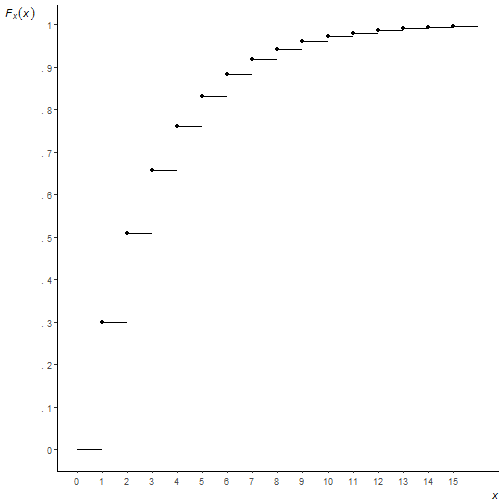You can see I couldn't figure out how to move the axis ticks into the plotting region, or how to place axis labels at the ends of the axis, or how to get the origin to start at precisely (0, 0). I'm not saying it can't be done, just that I lost interest in trying to go further. And a very strong argument can be made that these are things you shouldn't do anyway! But as I said at the outset, this was all for fun.

# Revisiting some old R-code

One of my first blog posts on this site simulated rolling a die 6 times and observing if side i was observed on the ith^ roll at least once. (For example, rolling a 3 on my 3rd roll would be a success.) I got the idea from one of my statistics textbooks which had a nice picture of the simulation converging to the true probability of 0.665 over the course of 500 trials. I was able to recreate the simulation in R and I guess I got excited and blogged about it. I was reminded of this today when I opened that old textbook and came across the R code that I had actually written in the margin by hand. Apparently I was super proud of my ground-breaking R code and decided to preserve it for posterity in writing. smh

Over 5 years later my precious R code looks pretty juvenile. It's way more complicated than it needs to be and doesn't take advantage of R's vectorized calculations. As Venables and Ripley say in MASS, “Users coming to S from other languages are often slow to take advantage of the power of S to do vectorized calculations…This often leads to unnecessary loops.” Indeed. I'm living proof of that.

I shouldn't be too hard on my myself though. I was not yet familiar with functions like replicate and cumsum. And I was more focused on recreating the plot than writing optimal R code. I went with what I knew. And R, so forgiving and flexible, accommodated my novice enthusiasm.

Here is how I would approach the problem today:

r.out <- replicate(n = 500, any(sample(1:6, size = 6, replace = T) == 1:6))
p.out <- cumsum(r.out)/seq(500)
plot(x = seq(500), y = p.out, type = "l", ylim = c(0,1),
main = "Convergence to probability as n increases", xlab = "n")
abline(h = 0.665)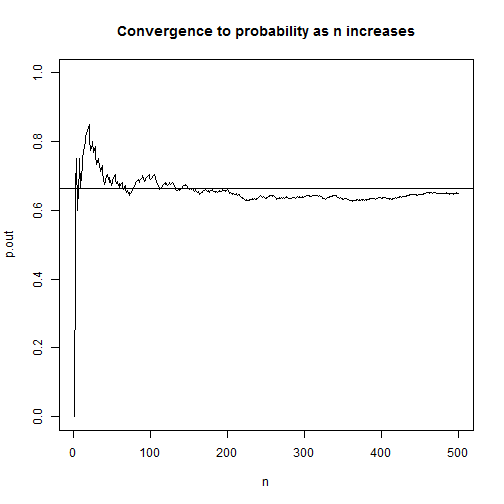On line 1, we use the sample function to “roll a die 6 times” by sampling the numbers 1 – 6, with replacement, 6 times. Then we compare the 6 results with the vector of numbers 1 – 6 using the == operator and use the any function to check if any are TRUE. Next we replicate that 500 times and store the result in r.out. This is a vector of TRUE/FALSE values which R treats numerically as 1 and 0. This means we can use cumsum to find the cumulative sum of successes. To determine the cumulative proportion of successes, we divide each cumulative sum by the trial number. The result is a vector of porportions that should start converging to 0.665. Finally we plot using base R plot and abline.

This is more efficient than my original attempt 5 years ago and better captures the spirit of the simulation. I'm sure 5 years from now if I stumble upon this post I'll have yet another more elegant way to do it. I'm already looking at it thinking, “I should have generalized this with a function, and used ggplot2 to make the graph. And I shouldn't do seq(500) twice.” In fact I know I could have avoided the replicate function by using the fact that there's a probablity of $$\frac{1}{6}$$ of observing side i on the ith^ roll of a die. So I could have used a single rbinom call to do the simulation, like so:

r.out2 <- cumsum(rbinom(n = 500, size = 6, prob = 1/6) > 0)
p.out2 <- r.out2/seq(500)
plot(x = seq(500), y = p.out2, type = "l", ylim = c(0,1),
main = "Convergence to probability as n increases", xlab = "n")
abline(h = 0.665)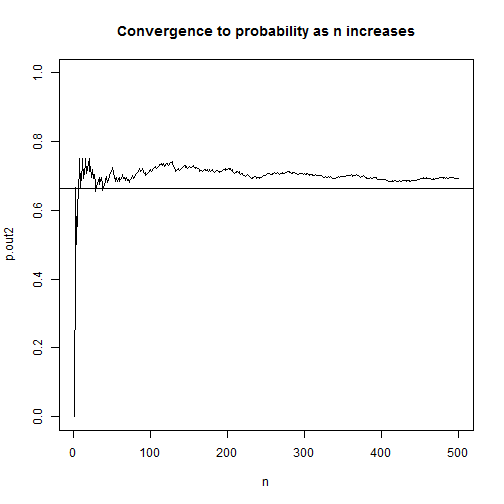In this version instead of simulating 6 literal die rolls, we simulate the number of successes in 6 die rolls. We turn each roll of the die into a binomial event: success or failure. The rbinom function allows us to simulate binomial events where size is the number of trials (or rolls in this case) and prob is the probability of success at each trial. So rbinom(n = 1, size = 6, prob = 1/6) would return a number ranging 0 to 6 indicating the number of success. Think of it as flipping 6 coins, each with probability of getting heads as $$\frac{1}{6}$$, and then counting the number of heads we observed. Setting the n argument to 500 replicates it 500 times. After that it's simply a matter of logically checking which outcomes were greater than 0 and using cumsum on the resulting TRUE/FALSE vector.

This version is way faster. I mean way faster. Compare the time it takes it to do each 1,000,000 times:

system.time({
r.out <- replicate(n = 1e6, any(sample(1:6, size = 6, replace = T) == 1:6))
p.out <- cumsum(r.out)/seq(1e6)
})

##    user  system elapsed
##    5.26    0.00    5.26

system.time({
r.out2 <- cumsum(rbinom(n = 1e6, size = 6, prob = (1/6)) > 0)
p.out2 <- r.out2/seq(1e6)
})

##    user  system elapsed
##    0.06    0.00    0.06


It's not even close. Who was the dummy that wrote that first version with replicate?

But does the new faster version reflect the experimental setting better? Not really. Remember, we're demonstrating probability concepts with die rolls in the first chapter of an intro stats textbook. That's probably not the best time to break out rbinom. And the demo was for 500 trials, not 1,000,000. I had to ramp up the trials to see the speed difference. Maybe the “right” R code in this situation is not the fastest version but rather the one that's easier to understand.

# Profile likelihood ratio confidence intervals

When you fit a generalized linear model (GLM) in R and call confint on the model object, you get confidence intervals for the model coefficients. But you also get an interesting message:

Waiting for profiling to be done...

What's that all about? What exactly is being profiled? Put simply, it's telling you that it's calculating a profile likelihood ratio confidence interval.

The typical way to calculate a 95% confidence interval is to multiply the standard error of an estimate by some normal quantile such as 1.96 and add/subtract that product to/from the estimate to get an interval. In the context of GLMs, we sometimes call that a Wald confidence interval.

Another way to determine an upper and lower bound of plausible values for a model coefficient is to find the minimum and maximum value of the set of all coefficients that satisfy the following:

$-2\log\left(\frac{L(\beta_{0}, \beta_{1}|y_{1},…,y_{n})}{L(\hat{\beta_{0}}, \hat{\beta_{1}}|y_{1},…,y_{n})}\right) < \chi_{1,1-\alpha}^{2}$

Inside the parentheses is a ratio of likelihoods. In the denominator is the likelihood of the model we fit. In the numerator is the likelihood of the same model but with different coefficients. (More on that in a moment.) We take the log of the ratio and multiply by -2. This gives us a likelihood ratio test (LRT) statistic. This statistic is typically used to test whether a coefficient is equal to some value, such as 0, with the null likelihood in the numerator (model without coefficient, that is, equal to 0) and the alternative or estimated likelihood in the denominator (model with coefficient). If the LRT statistic is less than $$\chi_{1,0.95}^{2} \approx 3.84$$, we fail to reject the null. The coefficient is statisically not much different from 0. That means the likelihood ratio is close to 1. The likelihood of the model without the coefficient is almost as high the model with it. On the other hand, if the ratio is small, that means the likelihood of the model without the coefficient is much smaller than the likelihood of the model with the coefficient. This leads to a larger LRT statistic since it's being log transformed, which leads to a value larger than 3.84 and thus rejection of the null.

Now in the formula above, we are seeking all such coefficients in the numerator that would make it a true statement. You might say we're “profiling” many different null values and their respective LRT test statistics. Do they fit the profile of a plausible coefficient value in our model? The smallest value we can get without violating the condition becomes our lower bound, and likewise with the largest value. When we're done we'll have a range of plausible values for our model coefficient that gives us some indication of the uncertainly of our estimate.

Let's load some data and fit a binomial GLM to illustrate these concepts. The following R code comes from the help page for confint.glm. This is an example from the classic Modern Applied Statistics with S. ldose is a dosing level and sex is self-explanatory. SF is number of successes and failures, where success is number of dead worms. We're interested in learning about the effects of dosing level and sex on number of worms killed. Presumably this worm is a pest of some sort.

# example from Venables and Ripley (2002, pp. 190-2.)
ldose <- rep(0:5, 2)
numdead <- c(1, 4, 9, 13, 18, 20, 0, 2, 6, 10, 12, 16)
sex <- factor(rep(c("M", "F"), c(6, 6)))
SF <- cbind(numdead, numalive = 20-numdead)
budworm.lg <- glm(SF ~ sex + ldose, family = binomial)
summary(budworm.lg)

##
## Call:
## glm(formula = SF ~ sex + ldose, family = binomial)
##
## Deviance Residuals:
##      Min        1Q    Median        3Q       Max
## -1.10540  -0.65343  -0.02225   0.48471   1.42944
##
## Coefficients:
##             Estimate Std. Error z value Pr(>|z|)
## (Intercept)  -3.4732     0.4685  -7.413 1.23e-13 ***
## sexM          1.1007     0.3558   3.093  0.00198 **
## ldose         1.0642     0.1311   8.119 4.70e-16 ***
## ---
## Signif. codes:  0 '***' 0.001 '**' 0.01 '*' 0.05 '.' 0.1 ' ' 1
##
## (Dispersion parameter for binomial family taken to be 1)
##
##     Null deviance: 124.8756  on 11  degrees of freedom
## Residual deviance:   6.7571  on  9  degrees of freedom
## AIC: 42.867
##
## Number of Fisher Scoring iterations: 4


The coefficient for ldose looks significant. Let's determine a confidence interval for the coefficient using the confint function. We call confint on our model object, budworm.lg and use the parm argument to specify that we only want to do it for ldose:

confint(budworm.lg, parm = "ldose")

## Waiting for profiling to be done...

##     2.5 %    97.5 %
## 0.8228708 1.3390581


We get our “waiting” message though there really was no wait. If we fit a larger model and request multiple confidence intervals, then there might actually be a waiting period of a few seconds. The lower bound is about 0.8 and the upper bound about 1.32. We might say every increase in dosing level increase the log odds of killing worms by at least 0.8. We could also exponentiate to get a CI for an odds ratio estimate:

exp(confint(budworm.lg, parm = "ldose"))

## Waiting for profiling to be done...

##    2.5 %   97.5 %
## 2.277027 3.815448


The odds of “success” (killing worms) is at least 2.3 times higher at one dosing level versus the next lower dosing level.

To better understand the profile likelihood ratio confidence interval, let's do it “manually”. Recall the denominator in the formula above was the likelihood of our fitted model. We can extract that with the logLik function:

den <- logLik(budworm.lg)
den

## 'log Lik.' -18.43373 (df=3)


The numerator was the likelihood of a model with a different coefficient. Here's the likelihood of a model with a coefficient of 1.05:

num <- logLik(glm(SF ~ sex + offset(1.05*ldose), family = binomial))
num

## 'log Lik.' -18.43965 (df=2)


Notice we used the offset function. That allows us to fix the coefficient to 1.05 and not have it estimated.

Since we already extracted the log likelihoods, we need to subtract them. Remember this rule from algebra?

$\log\frac{M}{N} = \log M – \log N$

So we subtract the denominator from the numerator, multiply by -2, and check if it's less than 3.84, which we calculate with qchisq(p = 0.95, df = 1)

-2*(num - den)

## 'log Lik.' 0.01184421 (df=2)

-2*(num - den) < qchisq(p = 0.95, df = 1)

##  TRUE


It is. 1.05 seems like a plausible value for the ldose coefficient. That makes sense since the estimated value was 1.0642. Let's try it with a larger value, like 1.5:

num <- logLik(glm(SF ~ sex + offset(1.5*ldose), family = binomial))
-2*(num - den) < qchisq(p = 0.95, df = 1)

##  FALSE


FALSE. 1.5 seems too big to be a plausible value for the ldose coefficient.

Now that we have the general idea, we can program a while loop to check different values until we exceed our threshold of 3.84.

cf <- budworm.lg$coefficients # fitted coefficient 1.0642 cut <- qchisq(p = 0.95, df = 1) # about 3.84 e <- 0.001 # increment to add to coefficient LR <- 0 # to kick start our while loop while(LR < cut){ cf <- cf + e num <- logLik(glm(SF ~ sex + offset(cf*ldose), family = binomial)) LR <- -2*(num - den) } (upper <- cf)  ## ldose ## 1.339214  To begin we save the original coefficient to cf, store the cutoff value to cut, define our increment of 0.001 as e, and set LR to an initial value of 0. In the loop we increment our coefficient estimate which is used in the offset function in the estimation step. There we extract the log likelihood and then calculate LR. If LR is less than cut (3.84), the loop starts again with a new coefficient that is 0.001 higher. We see that our upper bound of 1.339214 is very close to what we got above using confint (1.3390581). If we set e to smaller values we'll get closer. We can find the LR profile lower bound in a similar way. Instead of adding the increment we subtract it: cf <- budworm.lg$coefficients  # reset cf
LR <- 0                           # reset LR
while(LR < cut){
cf <- cf - e
num <- logLik(glm(SF ~ sex + offset(cf*ldose), family = binomial))
LR <- -2*(num - den)
}
(lower <- cf)

##    ldose
## 0.822214


The result, 0.822214, is very close to the lower bound we got from confint (0.8228708).

This is a very basic implementation of calculating a likelihood ratio confidence interval. It is only meant to give a general sense of what's happening when you see that message Waiting for profiling to be done.... I hope you found it helpful. To see how R does it, enter getAnywhere(profile.glm) in the console and inspect the code. It's not for the faint of heart.

I have to mention the book Analysis of Categorical Data with R, from which I gained a better understanding of the material in this post. The authors have kindly shared their R code at the following web site if you want to have a look: http://www.chrisbilder.com/categorical/

To see how they “manually” calculate likelihood ratio confidence intervals, go to the following R script and see the section “Examples of how to find profile likelihood ratio intervals without confint()”: http://www.chrisbilder.com/categorical/Chapter2/Placekick.R

# Earthquake data and Benford’s Law

Much has been written about Benford's Law, that weird phenonmenon where if you have a naturally occuring set of numerical data, 30% of the numbers will begin with 1, 18% will begin with 2, 12% will begin with 3, and so on. You might expect the distribution of leading digits to be uniformly distributed, but no, that just isn't the case. 60% of the time the leading digit is 1, 2, or 3. Though it may seem like some curious mathematical anomaly, Benford's Law has been used to detect fraud in elections and accounting.

In this post let's use R to verify that earthquake data obtained from the USGS does indeed follow Benford's Law. On May 28, 2016, I downloaded data for all earthquakes in the past 30 days. The data has a number of fields, including earthquake magnitude, depth of the earthquake, location, time and many others. Benford's Law says those numbers should follow a particular distribution.

The formula for Benford's Law is as follows:

$P(d) = \log_{10} \left(1+\frac{1}{d}\right)$

That says the probability that a digit d occurs as the first number is equal the log base 10 of 1 + 1/d. We can quickly generate these for d = 1 – 9 in R:

log10(1 + (1/(1:9)))

##  0.30103000 0.17609126 0.12493874 0.09691001 0.07918125 0.06694679
##  0.05799195 0.05115252 0.04575749


And we can make a quick plot as follows:

barplot(log10(1 + (1/(1:9))), names.arg = 1:9, main = "Benford's Law")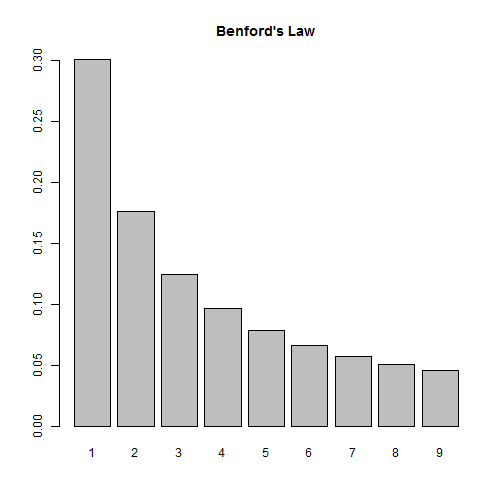So according to this law, if we look at the distribution of first digits in our earthquake data, we should see them closely follow this distribution. Let's find out!

First we need to import the data. Thanks to the USGS, this data comes ready for analysis. All we need to do is read it into R:

dat <- read.csv("all_month.csv")
nrow(dat)

##  8584


Over 8500 earthquakes in the past 30 days! A quick look at the magnitude shows us most of them are very small:

summary(dat$mag)  ## Min. 1st Qu. Median Mean 3rd Qu. Max. NA's ## -1.900 0.790 1.280 1.526 1.930 7.200 20  This also tells us we have some negative numbers (?) as well as some missing data, NA's. We also have numbers that start with 0 and have a decimal, such as 0.79. (In this case, Benford would say the number “starts” with a 7, not a 0.) This means when we determine the leading digits, we'll need to ignore negative signs, leading 0s, decimals and missing values. Let's investigate at the mag column. First we remove the NA's. Below is.na(dat$mag) generates a logical vector of TRUE and FALSE values. Adding an ! in front reverses the TRUE and FALSE values. Finally inserting the result in subsetting brackets returns only those values that are TRUE, ie not missing.

digits <- dat$mag[!is.na(dat$mag)]


Next we extract the first digits. To help with this I use two R packages, magrittr and stringr. The magrittr package allows us to “chain” commands together with the %>% operator. The stringr package provides the str_extract function that allows us to extract phrases that follow a certain pattern. So what we have below can be translated as follows:

• take the absoluet value of digits (to get rid of negative signs)
• convert digits to character (so we can use the next two functions)
• extract anything that is not a “0” or a “.” (We express this a regular expression: "[^0\\.]")
• extract the first digit (starting and ending at position 1)
library(magrittr)
library(stringr)
digits <- abs(digits) %>%
as.character() %>%
str_extract(pattern = "[^0\\.]") %>%
substr(1,1)


As an extra precaution, we then convert digits to a factor and set levels to be all digits 1 through 9. This ensure all digits are represented in subsequent calculations.

digits <- factor(digits, levels = 1:9) # ensure all digits represented


And finally we tally the first digits and calculate proportions:

table(digits) %>% prop.table()

## digits
##          1          2          3          4          5          6
## 0.42800141 0.17134139 0.05738763 0.09447248 0.05809177 0.03990142
##          7          8          9
## 0.04459570 0.05304542 0.05316277


We see 1 appearing a lot more often, but it's hard to tell how the other digits compare to Benford's Law. Let's put both distributions on the same graph. To do this, I went ahead and created a function that will allow us to check any vector of numbers against Benford's Law. Let's load the function and see how it works, then we'll break it down and explain it.

library(ggplot2)
compareBenford <- function(d){
digits <- d[!is.na(d)]
digits <- substr(stringr::str_extract(as.character(abs(digits)), pattern = "[^0\\.]"),1,1)
digits <- factor(digits, levels = 1:9) # ensure all digits represented
depth <- prop.table(table(digits))
ben <- log10(1 + (1/(1:9)))
dat2 <- data.frame(ben, depth)
names(dat2) <- c("Benford","Digit",deparse(substitute(d)))
dat2L <- reshape2::melt(dat2,id.vars="Digit", variable.name = "Type", value.name = "Frequency")
ggplot(dat2L, aes(x=Digit, y=Frequency, fill=Type)) +
geom_bar(stat = "identity", position = "dodge")
}

compareBenford(dat$mag)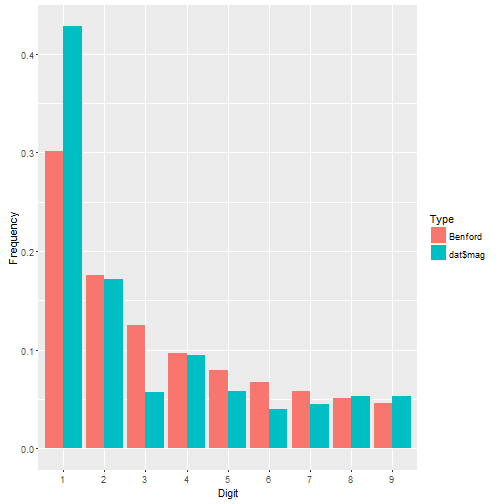We see dat$mag has more 1's than we might expect and fewer 3's, but otherwise seems to follow the distribution pretty closely. Let's check out earthquake depth.

compareBenford(dat$depth)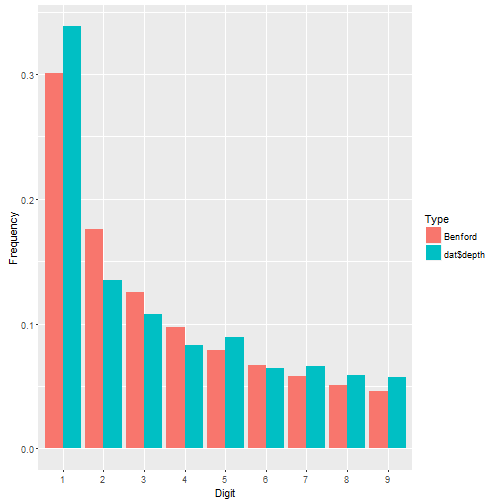This appears to fit even better. About the function: • the first four lines are what we did before. Notice I went ahead and nested the functions instead of using magrittr. That's how I originally coded it before deciding to write a blog post. Then I decided to break out magrittr for the fun of it. • After that I calculate Benford's proportions. • Then I put both sets of proportions in a data frame. • Then I change the names of the data frame. Notice there are three names to change, not two. Why? The depth vector is actually a table. When it gets pulled into a data frame, two columns are produced: the table cells and the table names. The names are the digits 1 – 9. Notice I also use deparse(substitute(d)) to name the first-digit proportions in the data frame. This ensures that whatever vector I give my function, the name of it will be the vector itself. So if I give it dat$mag, the column name of the first-digit proportions will be dat$mag. • Next I reshape the data using the melt function in the reshape2 package. This puts all the proportions in one column called Frequency and the type of proportion in another column called Type. I still have my Digit column that indicates which proportion goes with which digit. Having my data in this form allows me to use ggplot to map fill color to Type. • Finally I create the graph. I set Digit on the x-axis, Frequency on the y-axis, and map Type to the fill color. The geom_bar function says draw a bar plot. The stat = "identity" and position = "dodge" arguments say set the height of the bars to the actual values and “dodge” them so they're next to one another. Let's look at ALL numeric columns in the earthquakes data. We need to identify all numeric columns and pull them into a vector for our function to work. Here's how I do it: theCols <- sapply(dat, class) %in% c("numeric","integer") numCols <- names(dat)[theCols] allDigits <- unlist(dat[,numCols]) compareBenford(allDigits)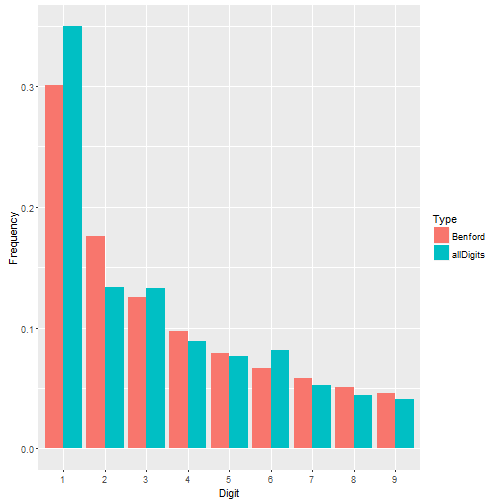Not a bad fit. Benford's Law seems to work just fine for the earthquake data. But what about data where Benford's Law doesn't work? Recall that Benford's Law applies to most naturally occuring sets of numbers. Not all. Take the iris data that come with R. These are measurements of 50 flowers from each of 3 species of iris. This is a small data set looking at very specific measurements. It doesn't seem to follow Benford's Law very well. irisDigits <- unlist(iris[,1:4]) compareBenford(irisDigits)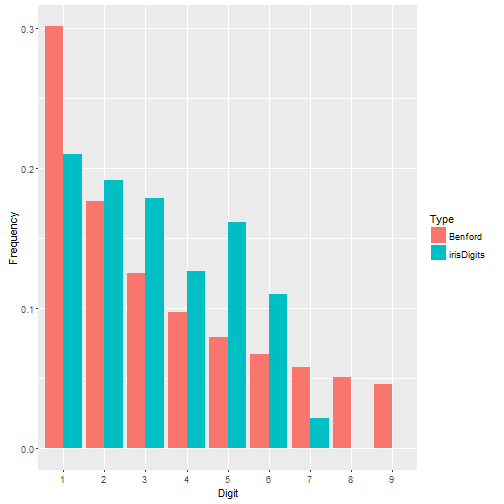# Recreating a graph from a NCHS data brief I was reading this sobering article about the rise of suicide rates in the United States and decided to read the NCHS data brief on which it was based. I noticed the source data was freely available online through the CDC Wonder databases, specifically the Detailed Mortality database, and figured I would take a look. One graph that caught my eye was Figure 2, which showed that suicide rates for females were higher in 2014 than in 1999 for all age groups under 75 years.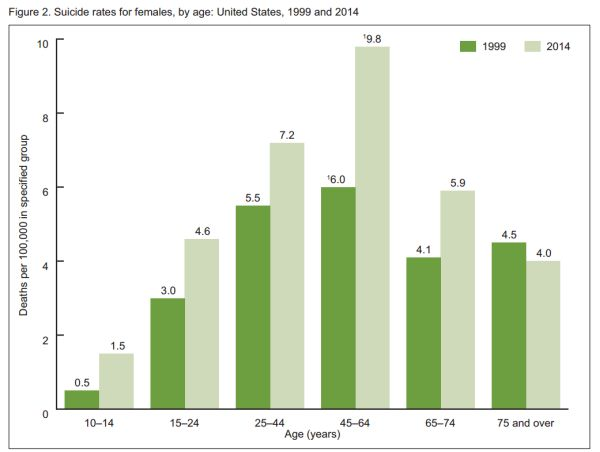That's a very sad and concerning graph. What happened in the last 15 years? I have no theories about the increase in suicide rates, but I do admire the CDC statisticians who tackle this kind of gut-wrenching data, day in and day out. Let's join them for a moment and recreate this graph in R. First we need to get the data from the Detailed Mortality database. Instead of directly downloading raw data, we submit a query for the data we want. There is a 7-step form to complete. Step 1: Group by ICD-10 113 Cause List, Cause of death, Age Groups, Gender, and Year. This uses all available pull-down lists. Step 2: leave as is. (ie, all US states) Step 3: leave as is. (ie, all genders, ages, and races) Step 4: select 1999 and 2014 using Ctrl + Click to select both years Step 5: leave as is. (ie, all weekdays, autopsies, and places of death) Step 6: select the ICD-10 113 Cause List radio button and select “Intentional self-harm (suicide) (*U03,X60-X84,Y87.0)” Step 7: click Send. The results should appear in your web browser. If everything looks good, click the Export button to download a tab-delimited file of the results. It will have a .txt extenstion. Now that we have our data, we can go to R and get to work. Before we read in the data we should open it in a text editor and see what we got. We have a header row, so that's good. If we scroll down, we'll see there's about 70 lines of metadata. This is data about our data, such as where it came from, when we downloaded it, etc. We don't want that in our data frame, but we should save it with our data frame as an attribute. To start we'll use readLines to read in the data one line at a time. I chose to do this as a way to programmatically figure out where the metadata begins. I noticed it begins after a divider of three dashes (“—”), so I use grep in combination with min to find the first occurence of this divider and save a n. raw <- readLines("Underlying Cause of Death, 1999-2014 (3).txt")  ## Warning in readLines("Underlying Cause of Death, 1999-2014 (3).txt"): ## incomplete final line found on 'Underlying Cause of Death, 1999-2014 ## (3).txt'  n <- min(grep(pattern = "---",raw))  Now I can use n with the nrows argument in the read.delim function to tell it how many rows I want to read in. Notice I have to say n - 2. I have to subtract the header row and the divider row itself. I also specify “Not Applicable” and “Unreliable” as NA values. Finally, take a look at my file name. Yep, it took me a few tries to get the data I wanted. I'm not too proud to admit that. :) dat <- read.delim(file = "Underlying Cause of Death, 1999-2014 (3).txt", header = TRUE, nrows = n-2, na.strings = c("Not Applicable","Unreliable"))  Let's go ahead and save the metadata with our data frame. We can do that with the comment function. We don't need it to create the graph, but it's good practice to preserve this kind of information. To see the metadata after we save, just enter comment(dat). It's not pretty when it's printed to the console, but we have it if we need it. comment(dat) <- raw[n:length(raw)]  Now we need to clean up the data. First order of business is to reorder the age groups. By default, R brought them in as a factor and put the 15-24 age group as the first level. We want that first level to be the 5-14 age group. It's already a factor, but we reorder the levels by making the column a factor again and specifying the levels in the specified order. The original 5th level comes first and then everything else. There may be a better way of doing this, but it works. dat$Ten.Year.Age.Groups.Code <- factor(dat$Ten.Year.Age.Groups.Code, levels = levels(dat$Ten.Year.Age.Groups.Code)[c(5,1,2,3,4,6,7,8,9,10)])


We only need females, so let's subset. I make a new data frame called dat2.

dat2 <- subset(dat, Gender=="Female")


Now if we look at Figure 2 again, the x-axis has 6 age groups, but our data has 9 age groups. So we need to combine a few age groups. Specifically we need to combine the “25-34” and “35-44” groups into “25-44”, combine the “45-54” and “55-64” groups into “45-64”, and the “75-84” and “85+” into “75+”. That's not too bad. We just reset the levels.

levels(dat2$Ten.Year.Age.Groups.Code) <- c("5-14", "15-24", "25-44", "25-44", "45-64", "45-64", "65-74", "75+", "75+", "NS")  Since we have 6 age groups instead of 9, we need to combine death totals for the three new age groups. We also need to combine death totals for all the various causes of deaths. We can do that with aggregate. Below we sum Deaths by Ten.Year.Age.Groups.Code and Year. This returns a new data frame that I call dat3. (dat3 <- aggregate(Deaths ~ Ten.Year.Age.Groups.Code + Year, data=dat2, sum))  ## Ten.Year.Age.Groups.Code Year Deaths ## 1 5-14 1999 50 ## 2 15-24 1999 575 ## 3 25-44 1999 2359 ## 4 45-64 1999 1868 ## 5 65-74 1999 420 ## 6 75+ 1999 469 ## 7 5-14 2014 151 ## 8 15-24 2014 990 ## 9 25-44 2014 3018 ## 10 45-64 2014 4195 ## 11 65-74 2014 828 ## 12 75+ 2014 477 ## 13 NS 2014 1  Next I need to calculate “deaths per 100,000” for each age group. We do this by dividing Deaths by the age group population and multiplying by 100,000. I need to add the age group populations to dat3 to do this. First I need to sum the populations for my new age groups. I can use aggregate again with dat2, but I need to remove duplicate rows of age group population values before I do this. I decided to save the de-duped data frame to tmp and not overwrite dat2 tmp <- subset(dat2, select = c("Year", "Ten.Year.Age.Groups.Code", "Population")) tmp <- tmp[!duplicated(tmp),] (dat4 <- aggregate(Population ~ Ten.Year.Age.Groups.Code + Year, data=tmp, sum))  ## Ten.Year.Age.Groups.Code Year Population ## 1 5-14 1999 19908282 ## 2 15-24 1999 18860184 ## 3 25-44 1999 42614694 ## 4 45-64 1999 31011068 ## 5 65-74 1999 10125424 ## 6 75+ 1999 10371906 ## 7 5-14 2014 20161424 ## 8 15-24 2014 21456371 ## 9 25-44 2014 41900194 ## 10 45-64 2014 42789506 ## 11 65-74 2014 14049245 ## 12 75+ 2014 11842674  Now I'm ready to merge dat3 and dat4 so I have Deaths and Populations in one data frame. Before I do that I remove the row with age group of “NS” which means “Not Stated”. We don't need that row. dat3 <- subset(dat3, Ten.Year.Age.Groups.Code != "NS") dat5 <- merge(dat3,dat4,by = c("Year","Ten.Year.Age.Groups.Code"))  And finally we can calculate Rate. I decided to also sort the data frame as well. dat5$Rate <- round(dat5$Deaths/dat5$Population * 100000,1)
(dat5 <- dat5[order(dat5$Ten.Year.Age.Groups.Code, dat5$Year),])

##    Year Ten.Year.Age.Groups.Code Deaths Population Rate
## 4  1999                     5-14     50   19908282  0.3
## 10 2014                     5-14    151   20161424  0.7
## 1  1999                    15-24    575   18860184  3.0
## 7  2014                    15-24    990   21456371  4.6
## 2  1999                    25-44   2359   42614694  5.5
## 8  2014                    25-44   3018   41900194  7.2
## 3  1999                    45-64   1868   31011068  6.0
## 9  2014                    45-64   4195   42789506  9.8
## 5  1999                    65-74    420   10125424  4.1
## 11 2014                    65-74    828   14049245  5.9
## 6  1999                      75+    469   10371906  4.5
## 12 2014                      75+    477   11842674  4.0


A quick comparison of our rates with those in Figure 2 shows that we're in agreement. Now it's time to re-create the graph. For this I chose to use ggplot2. To match the colors, I uploaded a picture of the original graph to this wonderful site and figured out the shades of green were #6D9F40 (dark green) and #CFDABA (light green).

library(ggplot2)
ggplot(dat5, aes(x=Ten.Year.Age.Groups.Code, y=Rate, fill=factor(Year))) +
geom_bar(stat="identity", position = "dodge") +
labs(x="Age (years)",y="Deaths per 100,000 in specified group") +
scale_fill_manual("Year", values = c("#6D9F40","#CFDABA")) +
annotate(geom = "text", x = (rep(1:6,each=2) + c(-1,1)*0.22),
y = dat5$Rate + 0.2, label = sprintf("%.1f", dat5$Rate)) +
theme_bw() +
scale_y_continuous(breaks=seq(0,10,2))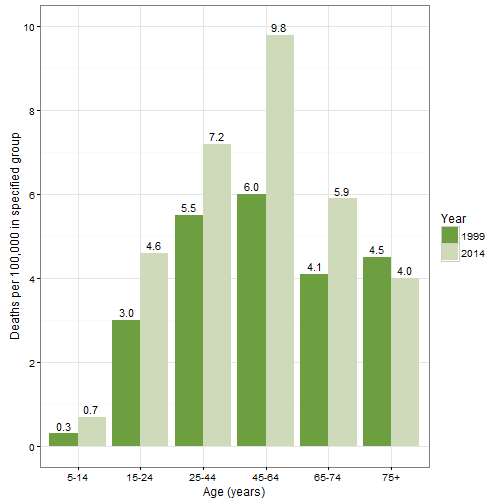The first line defines the aesthetics. I want Year to be treated like a categorical variable, so I call it with factor. The next lines says, “don't count the rows to create the bar graph, use the actual values of Rate (ie, identity).” It also says “dodge”“ the Years so they're next to each other (instead of stacked). The third line labels the axes. The fourth line titles the legend and defines the colors. The annontate line adds the counts to the plot. That took some trial and error! The x and y values are the coordinates. The label is the Rate values rendered as character with one decimal place. The theme_bw() line is an easy way to get rid of the default grey background and the last line relabels the y axis.

# Creating an R data package

A few weeks ago I got back into an old textbook of mine, Design and Analysis of Experiments (Dean and Voss). One of the things I love about this book is the incredible number of actual experiments used to demonstrate concepts. And all the data are available from the author’s website. My main interest in working through the book was to convert the many examples provided in SAS code into corresponding R code and to recreate the many plots. To do that meant having to download the data from the website and read it into R. Not that big of a deal, really, but I started thinking how nice it would be if the data were in an R package. Then I could just load the package and use the data at will. And I could document the data so I wouldn’t have to refer back to the book for variable definitions. And that’s what put me on the road to creating my first R data package, dvdata.

Now you’ll notice that last link went to GitHub, instead of CRAN. That’s because I asked one of the authors after I built the package if he would mind me uploading it to CRAN. Unfortunately, he did mind, because it turns out he’s working on his own R package for the next edition of the book. I was a little bummed, because I really wanted it on CRAN for the warm feeling of authenticity. But I understand. And besides the package still does what I wanted all along.

Now let’s talk about creating an R package. The very first thing you want to do is head over to Hadley Wickham’s R Packages site. He wrote a lovely book on how to create R Packages and posted it for free. And because it’s online, it almost always up-to-date. Hadley gently walks you through the process of creating an R package using his devtools package. I found it very easy to follow and I can’t recommend it enough.

What I want to do in this post is document in one place the basic steps to creating a R data package. All of these steps are in Hadley’s book, but they’re a little spread out due to the structure of the book, and because he covers a lot more than just making a simple data package.

Before you start, follow the directions under Getting Started in the Intro to Hadley’s book.

Steps to making an R data package

1. come up with a name for your package and create a package in RStudio as described here. This creates the smallest usable package. Let’s say you named your package “grrr”. On your computer you now have a directory called “grrr” which contains your package folders and files.

2. create two new directories: “data” and “data-raw” in your package directory.

3. go to the “R” directory in your package and delete the “hello.R” file.

4. Start a new R script called, perhaps, “get_data.R” and save to the “raw-data” directory. This will be the R script that reads in your data, wrangles it into shape and saves the data as an .RData file. You need to save the .RData objects into the “data” directory. The .RData objects are the data frames (or lists, or matrices, or vectors) your package will allow you to easily load. For examples, see Hadley’s R scripts in the “data-raw” directory of his babynames package.

5. When your data objects are done (i.e., the .RData files in your “data” directory) start an R script called “data.R” in the “R” directory. This is where you will compose the documentation for your data objects. Follow the directions in this section of Hadley’s book.

6. As you write your documentation, follow the The Documentation Workflow Hadley outlines in this section. Basically this involves submitting devtools::document() from the console and then previewing the documentation. Each time you submit devtools::document() Rd files are generated in the “man” directory of your package. (If you didn’t have a “man” directory, devtools creates one for you.) Do this until you are satisfied with your documentation.

7. Update the DESCRIPTION file as explained in this section. DESCRIPTION is just a text file.

8. Add ^data-raw$ to .Rbuildignore file. It too is just a text file. That keeps the “data-raw” folder from being included when the package is built. 9. Build the package: Ctrl + Shift + B. Feel free to do this at any point as you’re working on your package. That about does it! If you want to submit to CRAN, then read Hadley’s Release chapter very closely and follow it to the T. After creating dvdata, I created another data package called valottery that contains historical results of Virginia lottery drawings. This one I did get uploaded to CRAN. # A Note on Boxplots in R As a statistical consultant I frequently use boxplots. They're a great way to quickly visualize the distribution of a continuous measure by some grouping variable. More often than not, however, the person I'm helping doesn't regularly use boxplots (if at all) and is not sure what to make of them. Fortunately, boxplots are pretty easy to explain. The line in the middle of the box is the median. The box itself represents the middle 50% of the data. The box edges are the 25th and 75th percentiles. But what about the whiskers? That seems to be the part that trips people up. Before we go further, let's make some boxplots in R: set.seed(9) y <- rnorm(n = 100, mean = rep(c(22:25),each=25),sd = rep(c(5,8),each=50)) x <- gl(n=4,k=25) boxplot(y ~ x)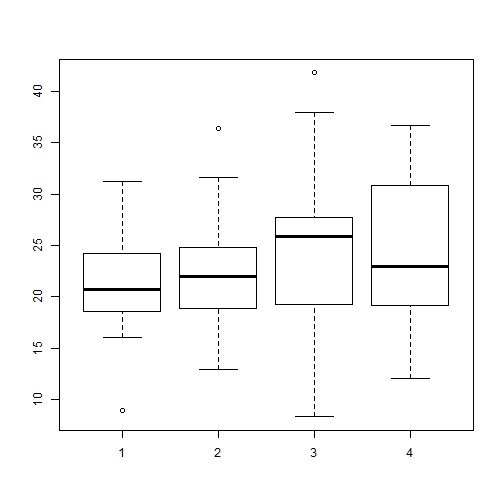Above I generate 100 random normal values, 25 each from four distributions: N(22,5), N(23,5), N(24,8) and N(25,8). Then I generate a 4-level grouping variable. Finally I make the boxplot. The black lines in the “middle” of the boxes are the median values for each group. For group 1, that appears to be a shade above 20. The vertical size of the boxes are the interquartile range, or IQR. They measure the spread of the data, sort of like standard deviation. The tops and bottoms of the boxes are referred to as “hinges”. We see groups 1 and 2 have less variability than groups 3 and 4, which makes sense given the way we generated the data. The IQR for group 1 looks to be about 5 judging from the height of the box. Again this makes sense given group 1 data was randomly generated from a normal distribution with a standard deviation of 5. And now we come to the “whiskers”, or the flattened arrows extending out of the box. What do they represent and how are they calculated? They represent the reasonable extremes of the data. That is, these are the minimum and maximum values that do not exceed a certain distance from the middle 50% of the data. What is that certain distance? By default in R, it's $$1.5 \times IQR$$. If no points exceed that distance, then the whiskers are simply the minimum and maximum values. That's the case in group 4. If there are points beyond that distance, the largest point that does not exceed that distance becomes the whisker. And the points beyond the distance are plotted as single points. For groups 1 through 3, there appear to be single points beyond the whiskers. (I say “appear to be single” because technically we could have overplotting.) We might think of these as outliers, data points that are too big or too small compared to the rest of the data. Group 4 does not appear to have outliers. When you create a boxplot in R, you can actually create an object that contains the plotted data. Just call the boxplot as you normally would and save to a variable. bp <- boxplot(y ~ x, plot = F) bp  ##$stats
##          [,1]     [,2]      [,3]     [,4]
## [1,] 16.06564 12.90309  8.300651 12.05522
## [2,] 18.53334 18.90152 19.281150 19.14307
## [3,] 20.75958 21.98459 25.924704 23.00494
## [4,] 24.18153 24.84778 27.721310 30.84133
## [5,] 31.22629 31.65432 38.016463 36.66171
##
## $n ##  25 25 25 25 ## ##$conf
##          [,1]     [,2]     [,3]     [,4]
## [1,] 18.97475 20.10557 23.25761 19.30830
## [2,] 22.54441 23.86361 28.59179 26.70159
##
## $out ##  8.911472 36.409950 41.843672 ## ##$group
##  1 2 3
##
## $names ##  "1" "2" "3" "4"  We see the bp object is a list with 6 different elements. The first element, stats, contains the plotted points in each group. So looking at column 1, we see that the bottom and top whiskers are 16.0656374 and 31.226286, the 25th and 75th percentiles are 18.5333406 and 24.1815345, and the median is 20.759577. The out element of the bp object contains the outliers while the group element contains the outliers' respective groups. If you want to change how whiskers are determined, you can change the range argument in the boxplot function. The default setting is 1.5. Here we try it set to 1: boxplot(y ~ x, range=1)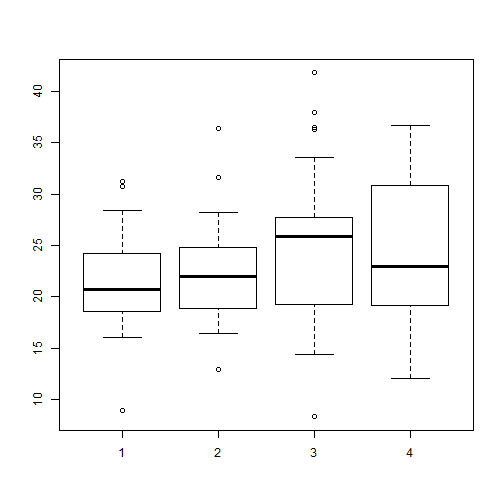Now we have more “outliers” for groups 1 – 3 but still none for group 4. Obviously the “outlier” classification is subjective. You'll also notice the size of the boxes didn't change. They will always capture the middle 50% of the data no matter the value of range. If we set range to 0, then the whiskers will extend to the minimum and maximum values for each group. In that case you get a plot of what is known as Tukey's Five-number summary: minimum, 25th percentile, median, 75th percentile and the maximum. In fact there's a function in R to calculate the Five-Number summary called fivenum. Here's how we can use it on our data: tapply(y,x,fivenum)  ##$1
##   8.911472 18.533341 20.759577 24.181534 31.226286
##
## $2 ##  12.90309 18.90152 21.98459 24.84778 36.40995 ## ##$3
##   8.300651 19.281150 25.924704 27.721310 41.843672
##
## $4 ##  12.05522 19.14307 23.00494 30.84133 36.66171  boxplot(y ~ x, range=0)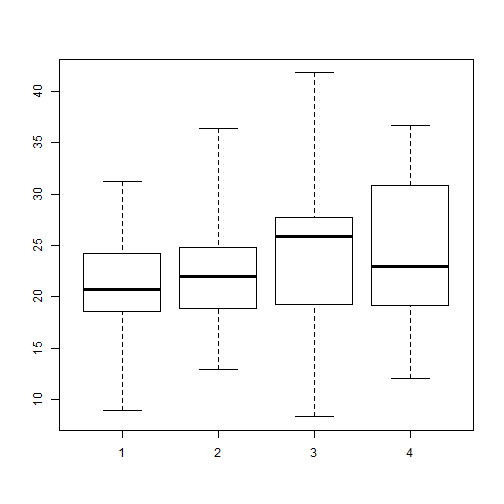A nice complement to boxplots are stripcharts. These are one-dimensional scatter plots of data. Here's out it works “out of the box”: stripchart(y ~ x)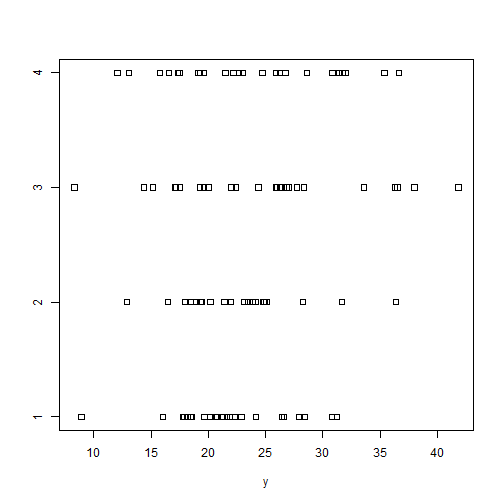I usually like to rotate stripcharts and use the familiar open circles with some “jitter”: stripchart(y ~ x, vertical = TRUE, pch=1, method="jitter")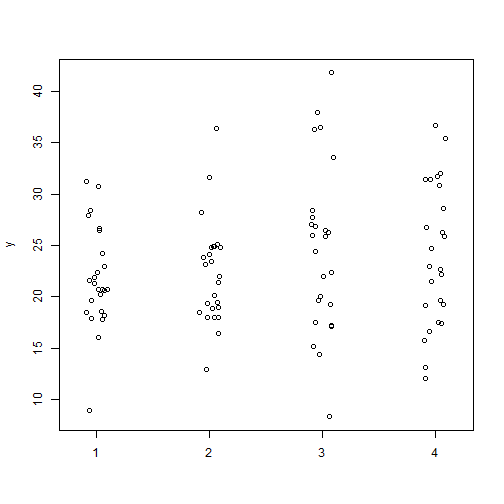Plotting stripcharts and boxplots side-by-side can be useful to visualize the spread and distribution of data. You can also calculate means and medians add them with the points function: op <- par(mfrow=c(1,2)) means <- tapply(y,x,mean) # calculate means medians <- tapply(y,x,median) # calculate medians boxplot(y ~ x, ylab="y") points(x = 1:4,y=means,pch=19) # add means stripchart(y ~ x, vertical = TRUE, pch=1, method="jitter") points(x = 1:4,y=means,pch=19, col="blue") # add means points(x = 1:4,y=medians,pch=19, col="red") # add means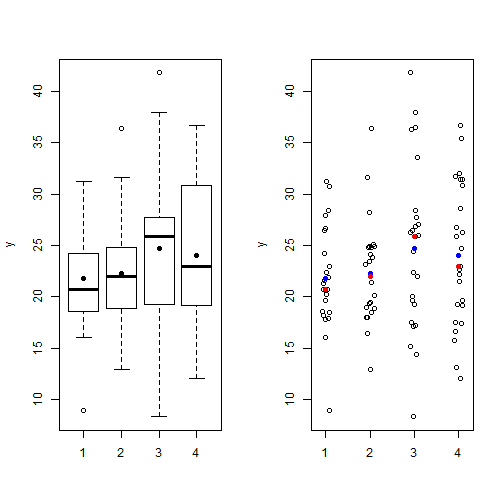par(op) # reset graphics parameters  # Poisson regression – Ch 6 of Gelman and Hill Chapter 6 of Gelman and Hill's Data Analysis Using Regression and Multilevel/Hierarchical Models presents an interesting example of Poisson regression using data on police stops in New York. Put simply, they seek to model the count of “stop and frisks” as a function of ethnicity and precinct with number of arrests in the previous year included as an offset. While the example is fun and informative, it's not terribly easy to replicate. The R code is provided to run the regressions but the data is a little hard to locate. I was eventually able to find it and get everything to mostly work, so I thought I would blog about it in case anyone else was trying to do the same. About the data: it turns out you can find it here in the “police” folder. In the folder is a dat file called “frisk_with_noise”. Here's one way to read it in: url <- "http://www.stat.columbia.edu/~gelman/arm/examples/police/frisk_with_noise.dat" dat <- read.table(url,header=TRUE, skip=6) head(dat,n=4)  ## stops pop past.arrests precinct eth crime ## 1 75 1720 191 1 1 1 ## 2 36 1720 57 1 1 2 ## 3 74 1720 599 1 1 3 ## 4 17 1720 133 1 1 4  dim(dat)  ##  900 6  But wait. You'll notice the data set has 900 rows but the regression output in the book references n = 225. To replicate the example in the book, I'm pretty sure we need to aggregate over precinct and eth, like so: stops <- aggregate(cbind(stops, past.arrests) ~ eth + precinct, data=dat, sum)  Using cbind() on stops and past.arrests allows us to sum both stops and past.arrests over all combinations of eth and precinct. Now we're ready to run the code provided in the book, which can also be found here. Here's one of the models they fit: library(arm) # for the display() function fit.2 <- glm (stops ~ factor(eth), data=stops, family=poisson, offset=log(past.arrests)) display(fit.2)  ## glm(formula = stops ~ factor(eth), family = poisson, data = stops, ## offset = log(past.arrests)) ## coef.est coef.se ## (Intercept) -0.59 0.00 ## factor(eth)2 0.07 0.01 ## factor(eth)3 -0.16 0.01 ## --- ## n = 225, k = 3 ## residual deviance = 45437.4, null deviance = 46120.3 (difference = 682.9)  That works, but our output doesn't match the book's output! What's going on? Actually the explanation is in the title of the data file: “frisk_with_noise”. Also notice how I skipped the first 6 lines of the file when reading it in to R. Here's the first line of what I skipped: stop and frisk data (with noise added to protect confidentiality)  So noise was apparently added after publication of the book to protect confidentiality. I guess we're not supposed to see which precincts are making the most stops of certain ethnicities? Oh well, at least we can run the code now. We can see fitted values using the fitted() function. Here are the first 10 fitted values compared to their observed values: cbind(observed=stops$stops[1:10], fitted=fitted(fit.2)[1:10])

##    observed    fitted
## 1       202  544.2816
## 2       102  175.7562
## 3        81  180.0316
## 4       132  418.2082
## 5       144  331.8515
## 6        71  203.6578
## 7       752 1215.1920
## 8       441  373.5563
## 9       410  584.9845
## 10      385  261.5884


Doesn't look too good to the eye, at least not the first 10. How are those fitted values calculated? Like this:

$y = exp(\alpha + \beta x + log(t))$

where t is the offset, in this case, past arrrests. So to calculate the fitted value for precinct 1, ethnicity = 1 (black), and 980 arrests in the previous year, we do the following:

exp(coef(fit.2) + log(980))

## (Intercept)
##    544.2816

# or equivalently
980*exp(coef(fit.2))

## (Intercept)
##    544.2816

# compare to R's fitted value
fitted(fit.2) == exp(coef(fit.2) + log(980))

##    1
## TRUE


Plotting standardized residuals versus fitted values can reveal overdispersion. That's what Gelman and Hill do in figure 6.1. Below I reproduce it. First I fit a new model that includes precinct as a predictor. The figure on the left plots raw residuals versus fitted values. This is of limited use since we expect variance to increase with larger fitted values in a Poisson distribution. In other words we wouldn't expect to see a constant scatter about 0 as we would in OLS regression. The figure on the right, however, uses standardized residuals which have a mean of 0 and standard deviation of 1. If the Poisson model is true, we would expect to see most residuals falling within 2 standard deviations from 0. Many residuals falling beyond this range reveal overdispersion. And indeed that's what we see here.

fit.3 <- glm (stops ~ factor(eth) + factor(precinct), data=stops, family=poisson, offset=log(past.arrests))
par(mfrow=c(1,2))
pv <- fitted(fit.3)
r <- (stops$stops - fitted(fit.3)) plot(pv, r, pch=20, ylab="raw residuals", xlab="predicted value") abline(h=0) sr <- (stops$stops - fitted(fit.3))/sqrt(fitted(fit.3))
plot(pv, sr, pch=20, ylab="standardized residuals", xlab="predicted value")
abline(h=c(-2,0,2),lty=c(2,1,2))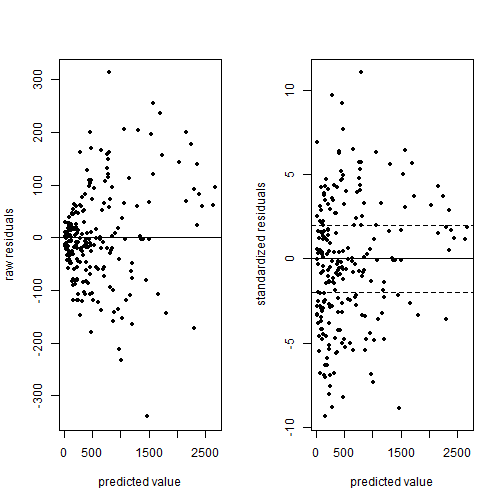par(mfrow=c(1,1))


To correct for overdisperson in R you can repeat the code for fit.3 above with family = quasipoisson. This corrects the standard errors of the regression coefficients by making them larger.

# Understanding Mosaic Plots

Mosaic plots provide a way to visualize contingency tables. However they’re not as intuitive as, say, a scatter plot. It’s hard to mentally map a contingency table of raw counts to what you’re seeing in a mosiac plot. Let’s demonstrate.

Here I use data from Example 8.6-3 of Probability and Statistical Inference (Hogg & Tanis, 2006). These data are a random sample of 400 University of Iowa undergraduate students. The students were classified according to gender and the college in which they were enrolled.

M <- matrix(data = c(21,14,16,4,145,175,2,13,6,4),
ncol=5,
dimnames = list(Gender=c("Male","Female"),
College= c("Business","Engineering","Liberal Arts","Nursing","Pharmacy")))

M
##         College
## Gender   Business Engineering Liberal Arts Nursing Pharmacy
##   Male         21          16          145       2        6
##   Female       14           4          175      13        4

To create a basic mosaic plot in R, you use the mosaicplot function with a contingency table as the first argument. This can be a table or matrix object. I’ve also added a title to the graph and used the las argument to make the axis labels horizontal.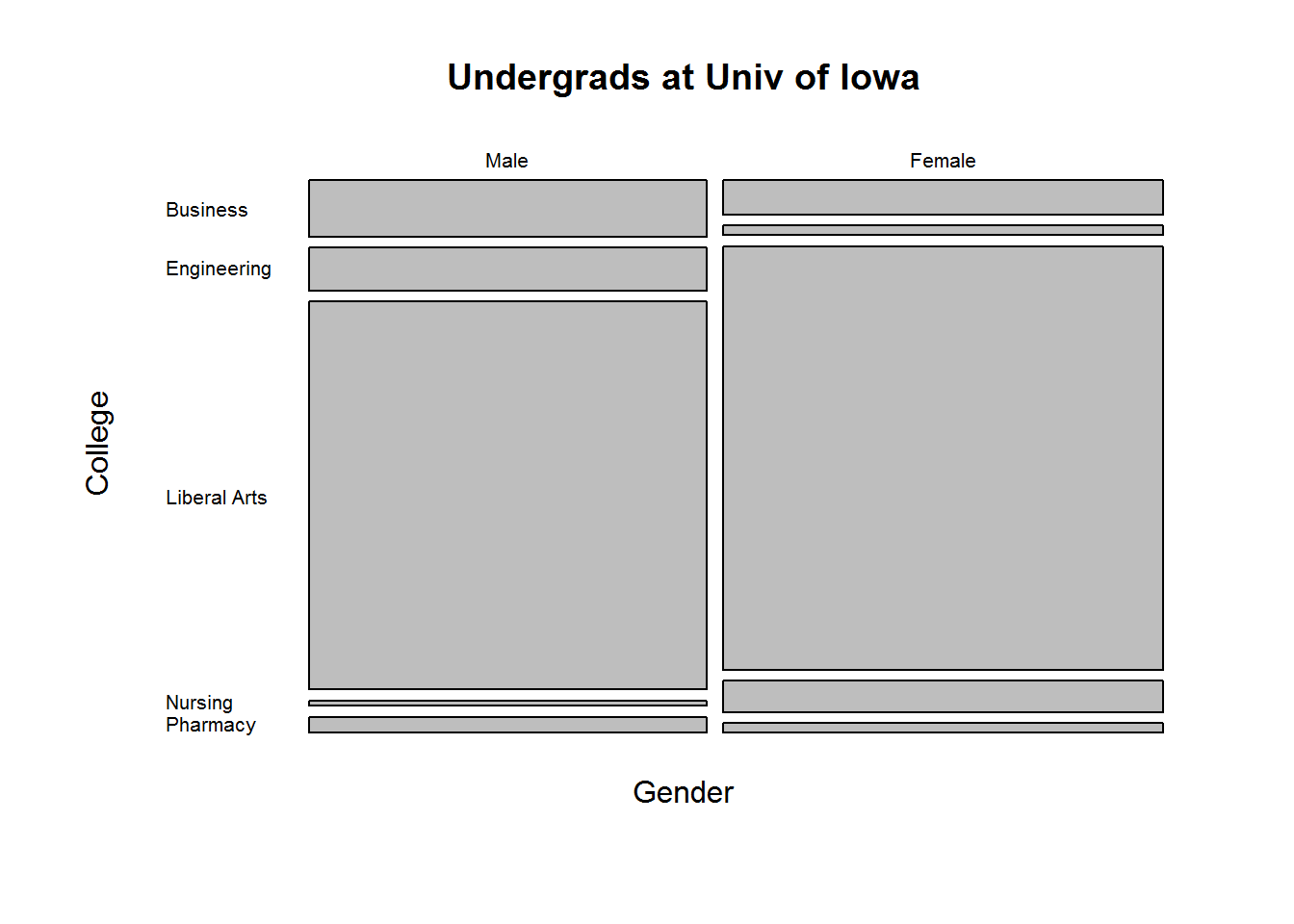The main feature that likely jumps out to you is the lack of numbers. We just see rectangles stacked on one another. If we compare the mosaic plot to the table of counts, the size of the boxes seem related to the counts in the table. Indeed they are, but how? Another feature you may notice is that the widths of the rectangles do not vary but the heights do. What’s up with that?

By default, the mosaicplot function recursively calculates marginal proportions starting with the rows. In our example, we start with gender:

apply(M, 1, function(x)sum(x)/sum(M))
##   Male Female
##  0.475  0.525

That simply says 0.475 of our sample is male, and 0.525 is female. These are the widths of the rectangles.

Now within gender, calculate the proportion belonging to each college:

prop.table(M, margin = 1)
##         College
## Gender     Business Engineering Liberal Arts    Nursing   Pharmacy
##   Male   0.11052632  0.08421053    0.7631579 0.01052632 0.03157895
##   Female 0.06666667  0.01904762    0.8333333 0.06190476 0.01904762

Among males about 11% are enrolled in the Business college versus only 6% among females. These are the heights of our rectangles.

The scale of the plot is basically 0 to 1 on the x and y axes. So the first rectangle we see in the Male column for the Business college is 0.475 wide and about 0.11 tall. In the Female column, the rectangle for the Business college is 0.525 wide and about 0.07 tall. Visually we see there are more Females than Males in our sample because the Female rectangles are wider. Within the gender columns, we see Males have a higher proportion in the Business school than do Females because their rectangle is taller.

That’s what mosaic plots attempt to visualize: recursive proportions of membership within a n-dimension table.

Let’s try it on a table with 3 dimensions. Below we’ll use a data set that comes with R called UCBAdmissions. This data set contains “aggregate data on applicants to graduate school at Berkeley for the six largest departments in 1973 classified by admission and sex.” This is a rather famous data set used for illustrating Simpson’s Paradox.

UCBAdmissions
## , , Dept = A
##
##           Gender
## Admit      Male Female
##   Admitted  512     89
##   Rejected  313     19
##
## , , Dept = B
##
##           Gender
## Admit      Male Female
##   Admitted  353     17
##   Rejected  207      8
##
## , , Dept = C
##
##           Gender
## Admit      Male Female
##   Admitted  120    202
##   Rejected  205    391
##
## , , Dept = D
##
##           Gender
## Admit      Male Female
##   Admitted  138    131
##   Rejected  279    244
##
## , , Dept = E
##
##           Gender
## Admit      Male Female
##   Admitted   53     94
##   Rejected  138    299
##
## , , Dept = F
##
##           Gender
## Admit      Male Female
##   Admitted   22     24
##   Rejected  351    317
mosaicplot(UCBAdmissions, main="Student Admissions at UC Berkeley")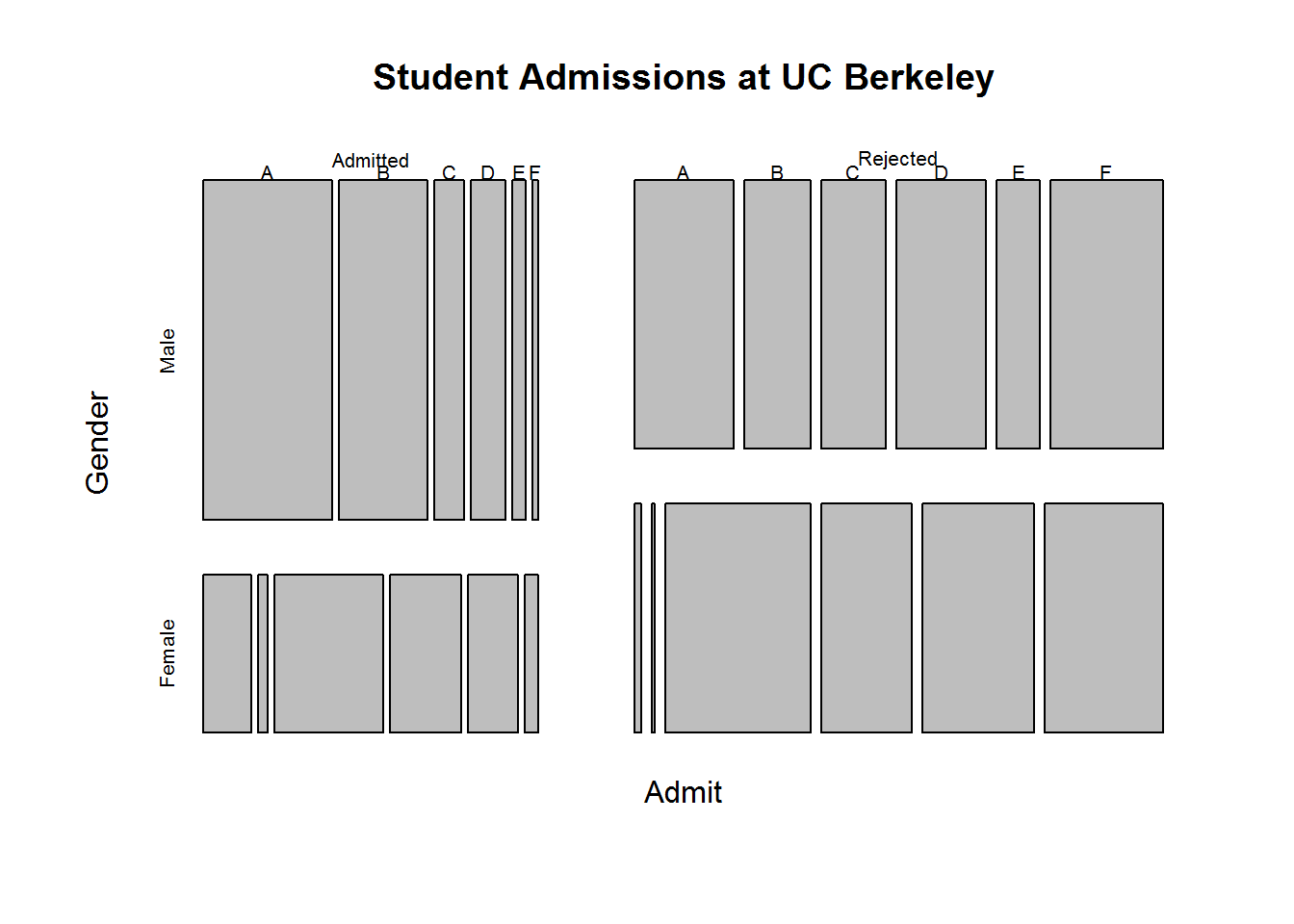How do we read this? Start with the Admit rows in our table of counts. That dictates the width of the two columns in the mosaic plot. Visually we see more people were rejected than admitted because the Rejected column of rectangles is wider. Next, go to the columns of the table: Gender. We see that of the people admitted, a much higher proportion were Male because of the height of the rectangles. Of the people rejected, it appears to be pretty even. Finally we move to the 3rd dimension: Dept. The height of these rectangles (or width, depending on how you look at it) is determined by proportion of Gender within Admit. So starting with the Admit column, compare the Dept rectangles between Male and Female. We see that a higher proportion of admitted Males were for Depts A and B compared to the proportion of admitted Females for the same Depts. On the other hand we see that a higher proportion of admitted Females were for Depts C – F compared to the proportion of admitted Males.

Were Depts A and B discriminating against Females? You might think so if you stop there. But look at the Rejected column. We see that of the rejected Males and Females, a much higher proportion of the Males were rejected for Depts A and B than Females. The widths of the Male rectangles are wider than their Female counterparts. Likewise for Depts C – F. It’s pretty clear that of the rejected Males and Females, a higher proportion of the Females were rejected for Depts C – F than Males. Again the widths of the Female rectangles are wider than their Male counterparts.

That’s where Simpson’s Paradox comes into play. If we disregard the within Dept counts, we see what appears to be Female discimination:

# collapse count over departments and create mosaic plot
margin.table(UCBAdmissions, margin = c(1, 2))
##           Gender
## Admit      Male Female
##   Admitted 1198    557
##   Rejected 1493   1278
mosaicplot(margin.table(UCBAdmissions, margin = c(1, 2)),
main = "Student admissions at UC Berkeley")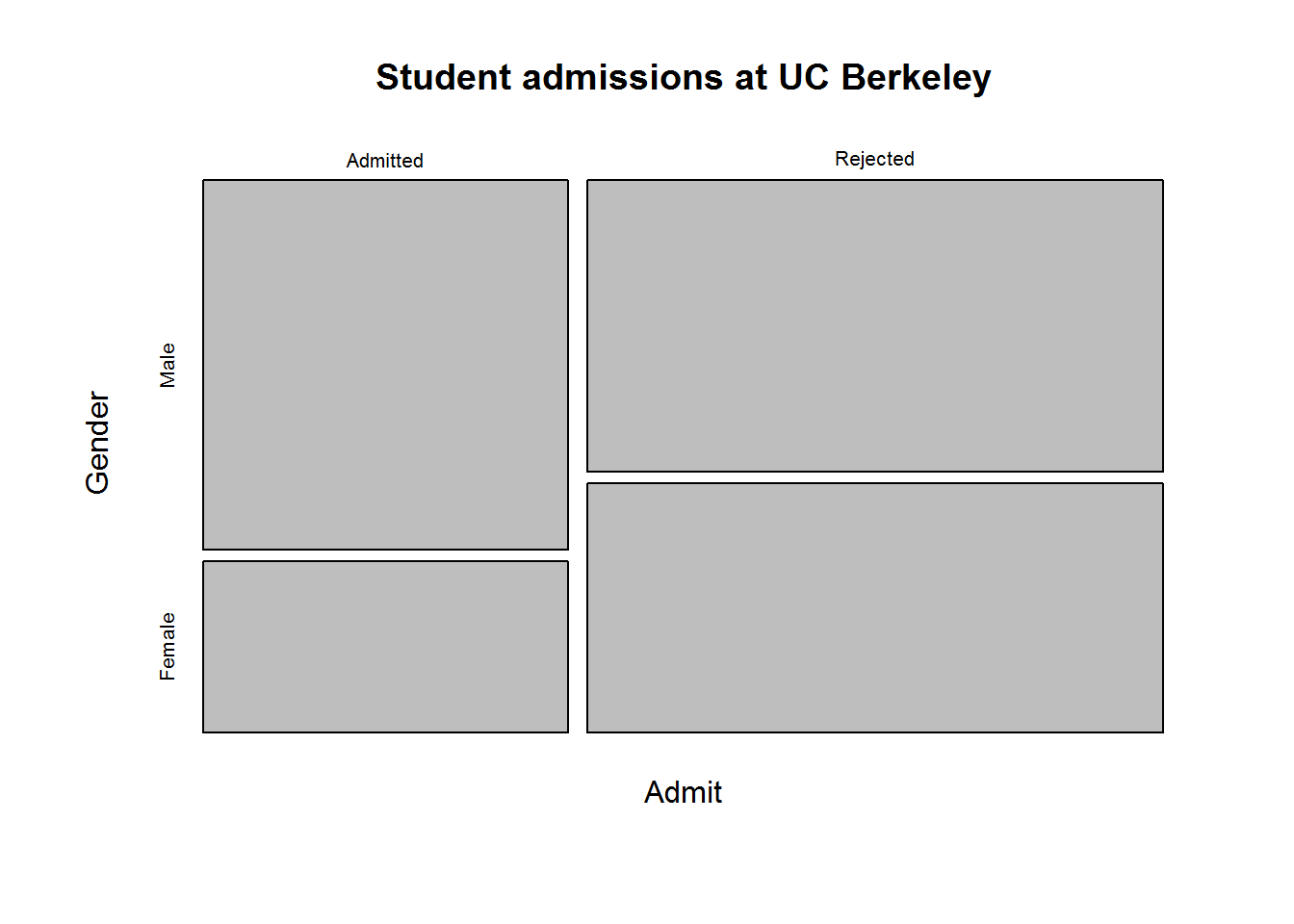To really understand what mosaic plots are showing, it helps to create one “by hand”. There’s no real point in doing so other than personal edification. But let’s be edified. We’ll work with our Univ of Iowa data.

We know our plot needs x and y axes with a scale of 0 to 1. We also know we need to draw rectangles. Fortunately R has a rect function that allows you to create rectangles. You tell it the coordinate points for the bottom left and upper right corners of your rectangle and it does the rest.

In order to translate the width and height of rectangles to locations within the plot, we’ll need to use the cumsum function. I need to draw rectangles relative to other rectangles. Hence the position of a rectangle corner will need to take into account other rectangles drawn above or beside it. The cumsum function allows us to do that.

Here’s my rough stab at a manual mosaic plot:

# widths
widths <- cumsum(c(0, apply(M, 1, function(x)sum(x)/sum(M))))
# heights
pt <- prop.table(M,margin = 1)
heightsM <- cumsum(c(0,pt[1,]))
heightsF <- cumsum(c(0,pt[2,]))
# Need to reverse the y axis
plot(x=c(0,1), y=c(0,1), xlim=c(0,1), ylim=c(1,0),
type = "n", xlab = "", ylab = "")
# male rectangles
rect(xleft = widths, ybottom = heightsM[-1],
xright = widths, ytop = heightsM[-6], col=gray(seq(3,12,2) / 15))
# female rectangles
rect(xleft = widths, ybottom = heightsF[-1],
xright = widths, ytop = heightsF[-6], col=gray(seq(3,12,2) / 15))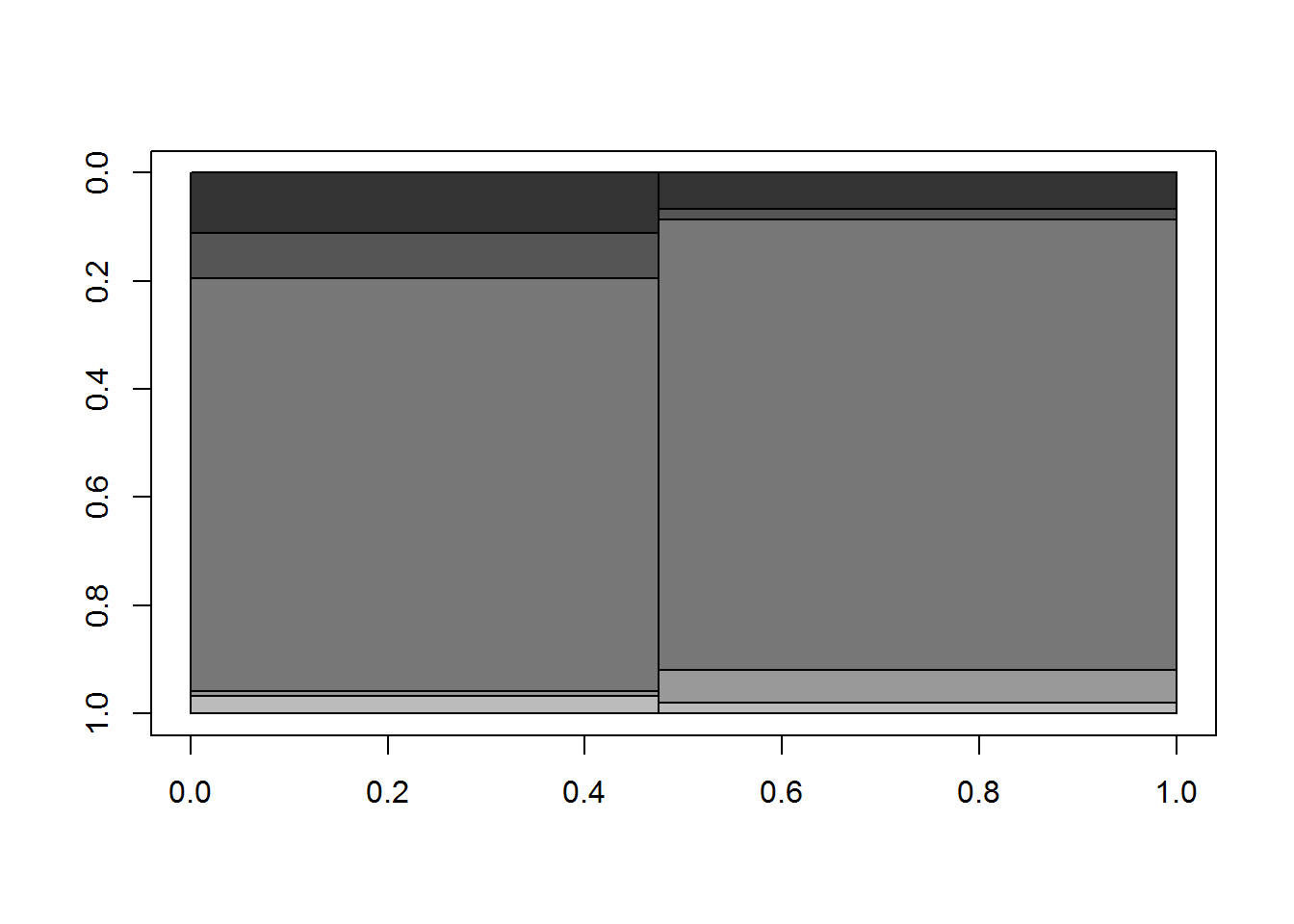If you compare that to the original mosaicplot() output above that I drew at the beginning of this post you can see we’ve basically drawn the same thing without spacing around the rectangles. That’s why I used the gray function to fill in the boxes with distinguishing shades. Again, nowhere near as nice as what the mosaicplot give us, but a good way to understand what the mosaicplot function is doing.